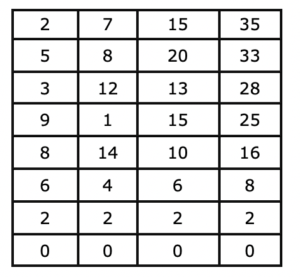# Four Number Challenge

Place any four numbers you choose in the first row of an array.

In the second row, in the first three columns write the difference of the two numbers just above and to the right in the first row (the larger minus the smaller). In the fourth column write the difference of the number above and the one in the first column of that row (again the larger minus the smaller). In other words, each entry is the absolute value of the difference of the two terms in the previous row.

Repeat for each row, in terms of the numbers in the row just above.

Will every choice of four number you begin with eventually lead to rows of zeros?

An Example: Begin with 2, 7, 15, 35Extension:

Find four beginning numbers that let you generate more than 10 rows with non-zero values. More than 20?